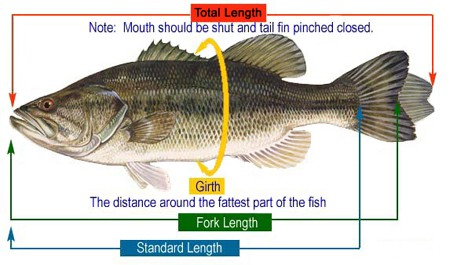## How to Estimate the Weight of a FishAs we all know, fishermen aren’t the best at making estimations when it comes to the weight of fish. Most overly exaggerate the size and weight of the fish caught, especially if it was released. So, i decided to help. I’m posting a fish weight estimation formula to help anglers get closer to the actual weight of the fish.To calculate the weight of a fish, you need to know two things: (1) The girth in inches and (2) the length in inches. The girth is the distance around the thickest part of the fish. The length should be measured from the tip of the lower jaw to the fork of the tail. If you don’t have a measuring tool, you can use pieces of fishing line cut to the length and girth. Then just measure the fishing line pieces.

Formula: First, you multiply the girth times the girth. Then multiply by the length. Then divide that sum by 800. This equals to the weight in pounds. [(Girth x Girth) x Length] / 800 = Weight in Pounds

While this estimation is pretty accurate, it’s still only an estimation. If you want the exact weight, then you will need to use a quality fishing scale. Save this page to your bookmarks/favorites, so you can come back to it when needed.